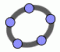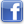Visualize - Practice - Understand successful learning at realmath.de
 Here are interactive dynamic worksheets according to Math Standards. Choose a section! ⇨Numbers   ⇨Algebra   ⇨Geometry   ⇨Measurement   ⇨Data Analysis and Probability

Numbers - Summary of worksheetsPlease note!
Dynamic constructions are created with GeoGebra.
 realmath.de facebookrealmath.de twitterTopic: Natural numbers Order of natural numbers Visualize Practice Hint - on the number line -1- ✓ ✓

 Topic: Fractions What's a fraction? Visualize Practice Hint - Create fractions ✓ ✓ - Identify fractions ✓ ✓ - Pouring juice in a mug ✓ ✓ - How much juice ...? ✓ ✓ - Determing fractions (meter) ✓ ✓ - Create fractions (meter) ✓ ✓ - Fractions of more wholes ✓ ✓ - Fill two boxes ✓ ✓ - Determing two boxes ✓ ✓ - Fractions of quantities ✓ ✓ - Fraction and time ✗ ✓ - Time and fraction ✗ ✓ - Fraction and quantity ✗ ✓ - Quantity and fraction ✗ ✓ - Determine the whole ✓ ✓ - Mixed number (tutorial) ✓ ✓ - Mixed number (exercise) ✗ ✓ - Improper fraction (tutorial) ✓ ✓ - Improper fraction (exercise) ✗ ✓ Arrange fractions Visualize Practice Hint - on the number line (1) ✓ ✓ - on the number line (2) ✓ ✓ - arrange four fractions -1- ✓ ✓ - arrange four fractions -2- ✓ ✓ - high or low? ✓ ✓ Rename fractions Visualize Practice Hint - Rename fractions (Tutorial) ✓ ✓ - Watch and rename ✓ ✓ - Find the missing number ✗ ✓ - Renamed correctly? ✗ ✓ - Find common denominators ✗ ✓ - Pearl necklet (part 1) ✓ ✓ Simplify fractions Visualize Practice Hint - Watch and simplify ✓ ✓ - Exercise ✗ ✓ - Simplified correctly? ✗ ✓ - Find common denominators ✗ ✓ - Pearl necklet (part 2) ✓ ✓ Compare fractions Visualize Practice Hint - compare with a half ✗ ✓ - High or low? ✗ ✓ - Between two fractions ✗ ✓ Add fractions (like denominator) Visualize Practice Hint - Tutorial and excercise ✓ ✓ - First exercise ✗ ✓ - Word problem ✓ ✓ - Magic square ✓ ✓ - Tricky exercise ✗ ✓ Add fractions (unlike denominator) Visualize Practice Hint - Tutorial and excercise ✓ ✓ - Exercise -part 1- ✗ ✓ - Exercise -part 2- ✗ ✓ - Exercise -part 3- ✗ ✓ Add mixed numbers Visualize Practice Hint - Integer and fraction (Tutorial) ✓ ✓ - Exercise level -1- ✗ ✓ - Exercise level -2- ✗ ✓ - Exercise level -3- ✗ ✓ Substract mixed numbers Visualize Practice Hint - Integer minus fraction ✓ ✓ - Mixed numbers minus fractions ✓ ✓ - Mixed numbers minus integers ✓ ✓ - Mixed numbers ✓ ✓ - Exercise level -1- ✗ ✓ - Exercise level -2- ✗ ✓ - Exercise level -3- ✗ ✓ Multiply fractions Visualize Practice Hint - Integer times fraction ✗ ✓ - Fraction times fraction -level 1- ✗ ✓ - Fraction times fraction -level 2- ✗ ✓ - Fraction times fraction -level 3- ✗ ✓ - Fraction times fraction (prof.) ✗ ✓ - Tricky exercise ✗ ✓ Divide fractions Visualize Practice Hint - Fraction diveded by fraction -level 1- ✗ ✓ - Fraction diveded by fraction -level 2- ✗ ✓ - Fraction diveded by fraction -level 3- ✗ ✓ - Fraction diveded by fraction (prof.) ✗ ✓ Mixed exercises Visualize Practice Hint - Computation tree -level 1- ✓ ✓ - Computation tree -level 2- ✓ ✓

 Topic: Integers Order of integers Visualize Practice Hint - number and opposite number ✓ ✓ - absolute value ✓ ✓ - compare integers ✓ ✓ - predecessor and successor 1 ✗ ✓ - predecessor and successor 2 ✗ ✓ Arrange integers Visualize Practice Hint - on the number line (1) ✓ ✓ - on the number line (2) ✓ ✓ - large integers on the number line (1) ✓ ✓ - large integers on the number line (2) ✓ ✓ Add integers Visualize Practice Hint - Add two integers ✗ ✓ - Fill the table ✗ ✓ - wall of numbers ✗ ✓ - What's the value of x? ✗ ✓ - tricky table ✗ ✓ - star with numbers ✗ ✓ - missing summand ✗ ✓ Substract integers Visualize Practice Hint - basic knowledge ✗ ✓ - Fill the table ✗ ✓ - wall of numbers ✗ ✓ - What's the value of x? ✗ ✓ Mixed exercises Visualize Practice Hint - add and substract (Tutorial) ✓ ✓ - add and substract level 1 ✓ ✓ - add and substract level 2 ✓ ✓ - add and substract level 3 ✓ ✓ - add and substract level 4 ✓ ✓ Multiply integers Visualize Practice Hint - wall of numbers level 1 ✗ ✓ - wall of numbers level 2 ✗ ✓ - wall of numbers level 3 ✗ ✓ - wall of numbers level 4 ✗ ✓ - wall of numbers -highlevel ✗ ✓

 Topic: Decimal numbers Place value Visualize Practice Hint - decimal and place value -level 1- ✗ ✓ new 06 - decimal and place value -level 2- ✗ ✓ new 06 - decimal and place value -prof.- ✗ ✓ new 06 - Text to decimals -level 1- ✗ ✓ - Text to decimals -level 2- ✗ ✓ - Text to decimals -level 3- ✗ ✓ - Recognize place values -level 1- ✗ ✓ - Recognize place values -level 2- ✗ ✓ - Recognize place values -level 3- ✗ ✓ Fraction and decimal fraction Visualize Practice Hint - Tutorial and exercise ✗ ✓ - Half, quater, eighth ✗ ✓ - Quater, fifth, tenth ✗ ✓ - Converting a fraction to a decimal ✗ ✓ - Decimal to fraction ✗ ✓ - Larger or smaller ✗ ✓ - Equivalent fractions ✗ ✓ Arrange decimal fractions Visualize Practice Hint - high or low? ✓ ✓ - Arranging tenths ✓ ✓ - Finding tenths ✓ ✓ - Arranging hundredths ✓ ✓ - Finding hundredths ✓ ✓ - Arranging tenth or hundredths ✓ ✓ - Finding tenth or hundredths ✓ ✓ - Arranging decimals part 1 ✓ ✓ - Finding decimals part 1 ✓ ✓ - Arranging decimals prof. ✓ ✓ - Finding decimals prof. ✓ ✓ - Between to decimals ✗ ✓ Add decimals Visualize Practice Hint - add two decimals ✗ ✓ - What's the value of x? ✗ ✓ - Complete the table (Part 1) ✗ ✓ - Complete the table (Part 2) ✗ ✓ - Tricky table ✗ ✓ - Wall of numbers (Part 1) ✗ ✓ - Wall of numbers (Part 2) ✗ ✓ - Decimalstar level -1- ✗ ✓ - Decimalstar level -2- ✗ ✓ - Find the missing summand ✗ ✓ Subtract decimals Visualize Practice Hint - substract two decimals (Part 1) ✗ ✓ - substract two decimals (Part 2) ✗ ✓ - Complete the table (Part 1) ✗ ✓ - Complete the table (Part 2) ✗ ✓ - What's the value of x? ✗ ✓ - What's the value of x? (Part 2) ✗ ✓ - Wall of numbers (Part 1) ✗ ✓ - Wall of numbers (Part 2) ✗ ✓ Multiply decimals Visualize Practice Hint - Multipy decimals by 10, 100, ... ✗ ✓ - Word problem ✗ ✓ Divide decimals Visualize Practice Hint - Divide decimals by 10, 100, ... ✗ ✓ - Word problem ✗ ✓

 Topic: Percentage Percentage and fractions Visualize Practice Hint - What's percentage? ✗ ✓ - Percentage and denominator 100 ✗ ✓ - Circle and rectangle (java) ✓ ✓ - Percentage and decimal numbers ✗ ✓ - Percentage and fractions ✗ ✓ - For professionals only (java) ✗ ✓

 Topic: Rational numbers Arrange rational numbers Visualize Practice Hint - high or low? ✗ ✓Support realmath.de! Please note, realmath.de is free for schools, students and all private users. Yet your donation could help covering the expenses of the development of these popular worksheets.
Disclaimer     Headmast   Notes on using this site     Data Protection   Contact      © Andreas Meier